# GMAT Math : DSQ: Calculating the surface area of a cube

## Example Questions

### Example Question #1 : Dsq: Calculating The Surface Area Of A Cube

Ron is making a box in the shape of a cube. He needs to know how much wood he needs. Find the surface area of the box.

I) The diagonal distance across the box will be equivalent to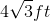.

II) Half the length of one side is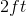.

Statement II is sufficient to answer the question, but Statement I is not sufficient to answer the question.

Both statements are needed to answer the question.

Either statement is sufficient to answer the question.

Statement I is sufficient to answer the question, but Statement II is not sufficient to answer the question.

Either statement is sufficient to answer the question.

Explanation:

To find the surface area of a cube, we need the length of one side.

Statement I gives the diagonal, we can use this to find the length of one side.

Statement II gives us a clue about the length of one side; we can use that to find the full length of one side.

The following formula gives us the surface area of a cube: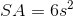Use Statement I to find the length of the side with the following formula, whereis the diagonal andis the side length: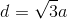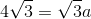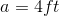So, using Statement I, we find the surface area to be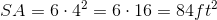Using Statement, we get that the length of one side is two times two: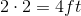Again, use the surface area formula to get the following:Tired of practice problems?

Try live online GMAT prep today.